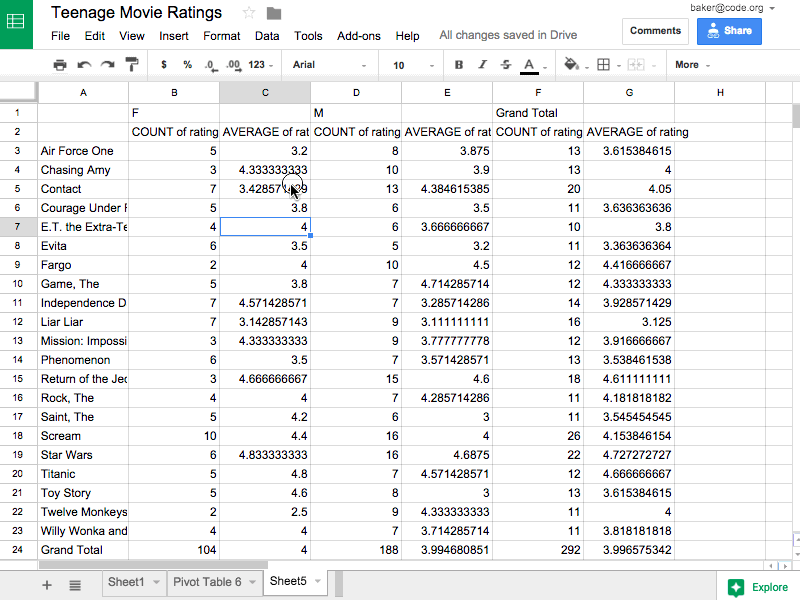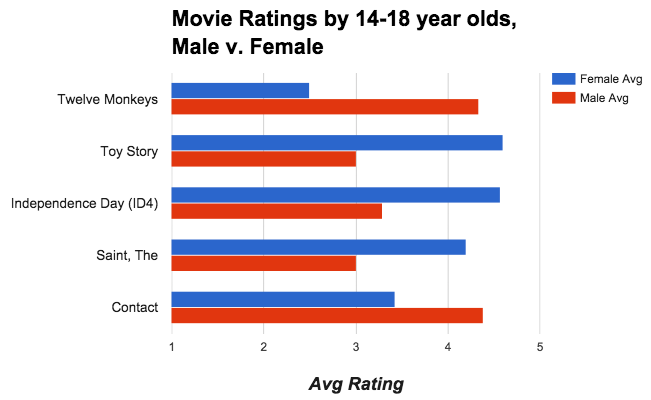# *Summary Tables Tutorial

This tutorial will take you through making summary tables. A summary table is a new spreadsheet that instead of having all of the data, has new data that has statistics computed from the original data. See the Data Statistics Chapter of the wikibook for a discussion of some of the data statistics that you can use in summary tables.

### Copy Data

To start, make a copy of Teenage Movie Ratings Subset.csv and open it in a spreadsheet program.

• The data is a subset of the larger movie ratings dataset we saw in a previous lesson.
• The dataset contains roughly 300 movie ratings that were collected online in 1997-98.
• The data has been filtered so that it only contains movies that were rated by at least 2 females and 2 males in the 14-18 year-old range.

### Making Summary Tables

We're first going to make a simple summary table that shows the average rating for every movie that's in the data. Here is what we're going for:In most spreadsheet programs a Summary Table is called a pivot table.
Do This: With the spreadsheet open in Google Sheets choose * Data -> Pivot table... *

The menu on the right side of the pivot table lets you choose what you want the rows, columns, and values to be in your summary table. We want to set it up so that:

• Each row is one movie.
• Each value is the average rating of that movie.
Do This: Follow the animation below to make a table that displays the average rating for every movie listed in the data set.

### What Happened? Computation!

The power of the pivot table is that it allows you to compute things you could never do by just filtering and sorting. The pivot table is doing a lot of computing behind the scenes for you - which is great - but you should understand what's really happening so you can make your own choices in the future. Here's a synopsis:

• Rows - Group By: movie
• Rows act like the major categories or groupings for which you want to calculate values.
• The Computation: When you set the rows to be "movie," the software finds all of the unique movie titles in the raw dataset and puts one on each row. This is called aggregation, which is a fancy word that means grouping or clustering.
• Values - Display: rating; Summarize by: AVERAGE
• Values lets you specify the computation that should happen for each row.
• The Computation: We're interested in the average rating for each movie, so for Values we choose rating, Summarize by: AVERAGE.

### Let's Change the Value -- Summarize by: COUNT

Change summarize by from AVERAGE to COUNT. Now, instead of computing the average rating, this will count the number of ratings for each movie.

### Add Another Field To Values

Let's show both the average rating and the count side-by-side in the table. To do this we add another Values field. The count is already there, so let's add the average rating again. Now, for each movie we'll see the total number of ratings the average rating.

### Summary - Basics of Pivot Tables

There's not much more to it than that. Once you get the hang of pivot tables they can be a very powerful tool for manipulating data. There are more advanced things you can do with a pivot table if you like, but you know enough now that you can probably just play around with the other settings and see what happens.
Key Ideas:

• Summary tables (pivot tables) provide a way to visualize data. Yes, it's a table, but by aggregating and summarizing information from a large data set, summary tables allow you to see things in the data you might otherwise not see.
• Summary tables allow you to manipulate and create new data. Even for our simple movies example here, the raw data didn't contain the average rating for every movie, or count how many ratings there were. We had to compute it, and the pivot table let us do that quickly and easily.
• A summary table helps you look at your data in new ways. Think: how could data be grouped? What could be calculated? Once you know how to make a summary table you can begin to look at raw data and ask questions that you know might be possible to answer.
• A summary table can be a first step toward a good visualization. Often it's difficult to make a meaningful chart or graphic out of raw data. You often want to summarize it first, then chart it!

Let's look at two more features of pivot tables that will allow you to do more complex investigations of your data. We learned that a Row in a pivot table specifies an aggregation or grouping of items for which you want to compute a value. A Column in a pivot table is just another aggregation, but it displays the values across the top of the table. It's easier to understand when you see it...
Do This: Add columns that group your data by gender, as in the animation below.

The image above show adding columns grouped by gender. The resulting table shows the average rating and count for each movie, but also broken down by gender. The pivot table also preserves the "Grand Totals" which is what the data would look like if no columns were specified.

### Filtering Pivot Tables

Applying a filter to a pivot table does the same thing as it does in the normal spreadsheet - it allows you to filter out values from the raw data. The animation below shows first filtering out 14-year-olds from the calculations, and then filtering out some of the movies. You don't have to do this, but in some instances it can be a very useful tool.

### Manipulating The Pivot Table

If you want to maninpulate the data further, to sort or filter, you shouldn't do it in the live, active pivot table. Instead you should copy the table, and paste the values into a new spreadsheet. Note: "Paste Values" is not the same as a normal "Paste".
Do This: Copy the pivot table, create a new tab in the spreadsheet and do Edit -> Paste special -> Paste values only. Watch the animation to see how.

The above images shows copying a pivot table, making a new tab in the spreadsheet, and pasting values. "Why Paste values only instead of just Paste?"

• If you copy a pivot table and do a normal paste it will paste another copy of the active, live, responsive pivot table into a new tab. We don't want the active table; we just want the values it produced.
• You probably want to add/change column headings to display the table, especially to use it for charting. See the image below for how you might do this.The above image shows changing column names to make them easier to read for a chart. From cleaning that up, plus some filtering we can make a chart of movies where the differences between male and female ratings are significant.### Visualizing Summary Tables

A summary table can be a good first step toward a great visualization. You often want to summarize data first, then chart it, so you can see larger connections or patterns. Summary tables also don't have to be small! You might make a summary table that is still too big or full of numbers to see any trends in the data. For example, from the original movie rating data (which had roughly 65,000 records) if you make a pivot table that shows the average movie rating for every possible age group the table will be about 75 rows long with a whole bunch of decimal numbers. You can't see any trend or pattern in the data just by looking at the table. But if you plot the results on a graph you can!NOTE: A deeper investigation of the data shows that the number of movies rated by people at this web site declined steadily after age 28. The upward trend may be affected by the fewer number of ratings.

* Wording, concepts, and materials for parts of this tutorial were taken from educational material provided by code.org under the Creative Commons Attribution-NonCommercial-ShareAlike 4.0 Unported License .